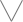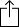C♭
D♭
E♭
F♭
G♭
A♭
B♭
Chord
Scale
3nps
CAGED
None
Notes
Degrees
Intervals
None

1
2
3
4
5
6
7
8
9
10
11
12
13
14
15
16
17
18
19
20
21
22
23
24
E
B
G
D
A
E
B
F
G
A
A
B
B
C
C
D
D
E
F
F
G
A
A
B
B
C
C
D
D
E
F
C
D
D
E
F
F
G
A
A
B
B
C
C
D
D
E
F
F
G
A
A
B
B
C
A
B
B
C
C
D
D
E
F
F
G
A
A
B
B
C
C
D
D
E
F
F
G
A
E
F
F
G
A
A
B
B
C
C
D
D
E
F
F
G
A
A
B
B
C
C
D
D
B
C
C
D
D
E
F
F
G
A
A
B
B
C
C
D
D
E
F
F
G
A
A
B
F
G
A
A
B
B
C
C
D
D
E
F
F
G
A
A
B
B
C
C
D
D
E
F
C
D
D
E
F
F
G
A
A
B
B
C
C
D
D
E
F
F
G
A
A
B
B
C

# Eb Super Locrian for 7 String Guitar

Eb Super locrian, sometimes called "Eb Diminished Whole Tone" is a diatonic scale and is the 7th mode in the set of Melodic minor modes

E
F
G
A
B
C
D
EH
W
H
W
W
W
W
Play scale
Install fretastic on your iPhone:
tapand then
"Add to homescreen"